Return to Free Library

Return to Number Menu

In this article we will take a look at the quintessential symbol of the Triad: the triangle. It will take three articles to explain many of the fascinating and important aspects of triangles.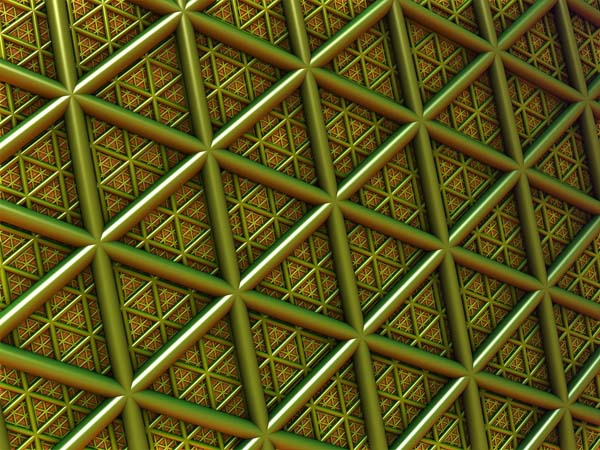# Triangles – 180º

Triangles have three sides and three angles.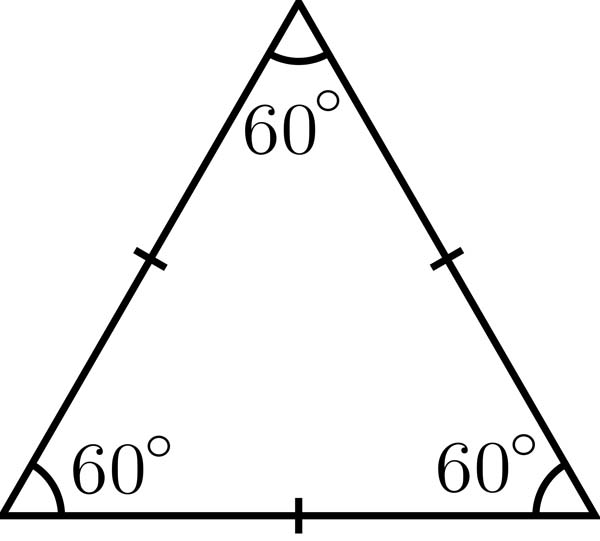All angles in a triangle always add up to 180 degrees. (A + B + C = 180)

180 hertz = F#. Therefore the triangle is inherently related to the F# note.

The area of a triangle equals ½ the base x the height:

A = ½ bh (A = bh/2)

A = area; b = base; h = height (measured at right angles to the base)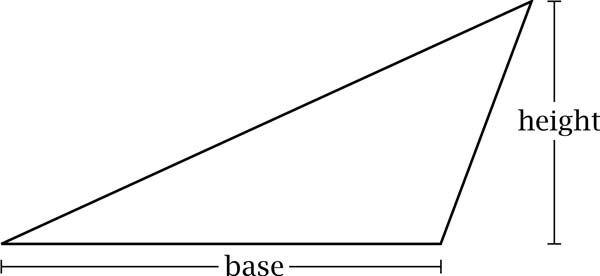#### Parts of a triangle:

• base – the horizontal side below the rest of the triangle
• apex – the vertex (corner) opposite the base
• altitude – a line drawn perpendicular to the base up to the apex – signifies the height of the triangle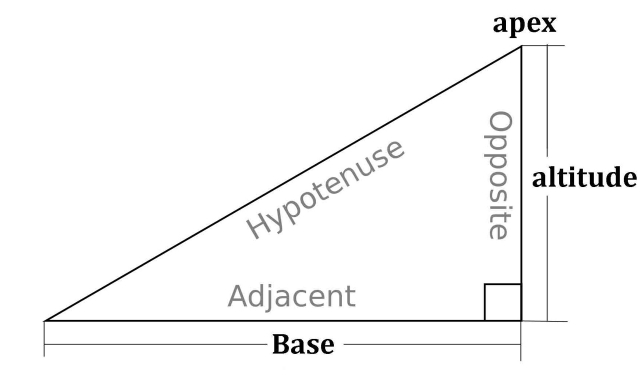A triangle can have three possible altitudes. An altitude of a triangle is a line drawn from any vertex perpendicular to the opposite side. These are shown in red, blue and green.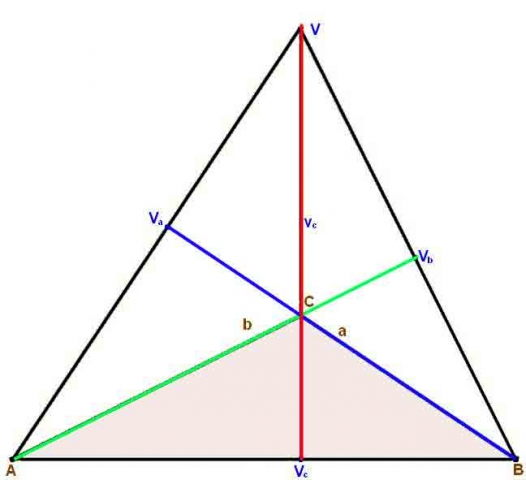The triangle is the most astringent shape.

It is the definition of structure. It is the only polygon structurally rigid by virtue of its geometry alone.A rectangle can collapse into a parallelogram from pressure to one of its points.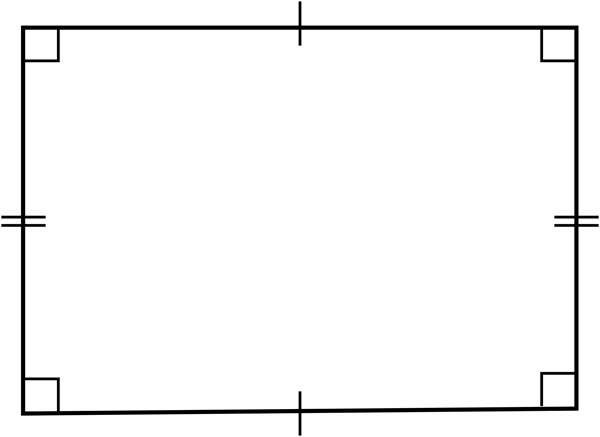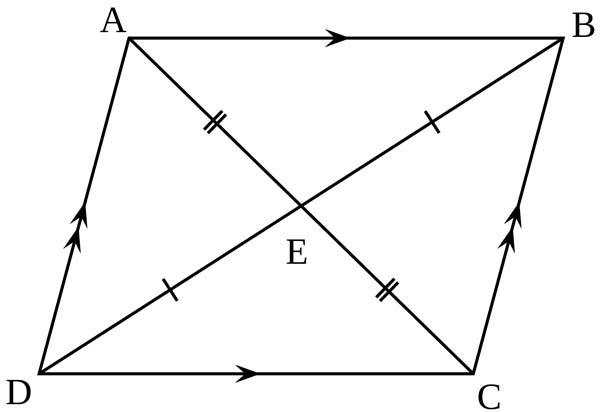Triangles have a natural strength which supports structures against lateral pressures.

A triangle will not change shape unless one of its sides are bent or extended or broken, or if its joints break. Each of the three sides supports the other two.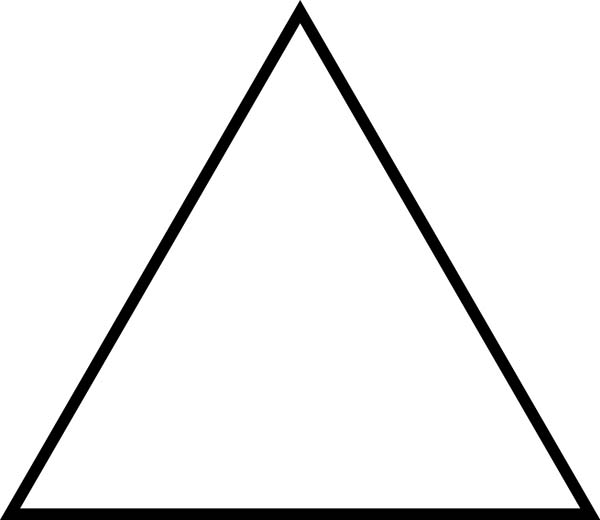A rectangle, on the other hand, is more dependent upon the strength of its joints.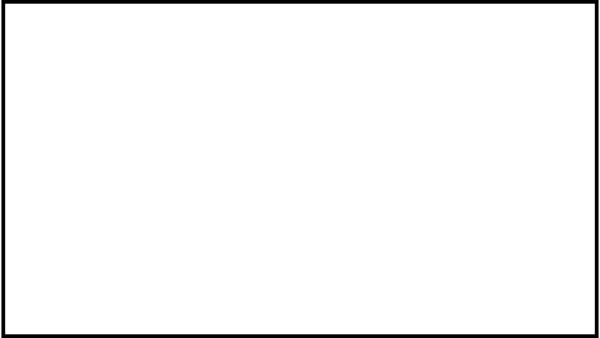The triangle’s stability and superior strength are unpredicted by any of its parts, which by themselves, do not have these properties. It is the three lines together that create the balance.It is self-sufficient. It resolves opposite tensions into one solid stable whole needing no support from without.

The triangle encloses the smallest area for the greatest perimeter.

This is opposite to the circle which encloses the greatest area with the smallest perimeter.

Equilateral triangles form the faces of three Platonic solids: tetrahedron, octahedron, and icosahedron.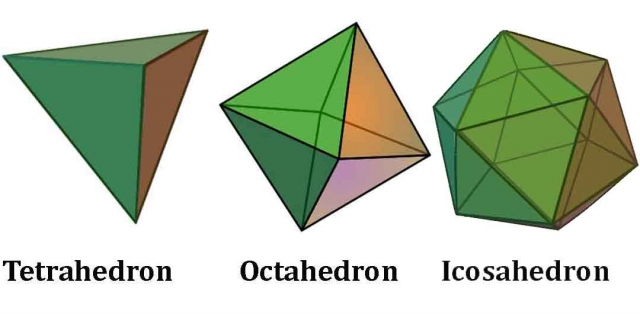There are three polygonal tessellations: triangles, hexagons and squares.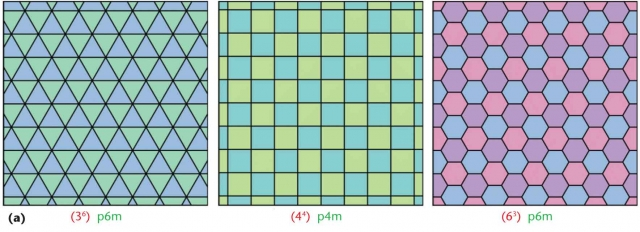Triangles are strong, but while packed in a tessellating arrangement they are not as strong as hexagons under compression.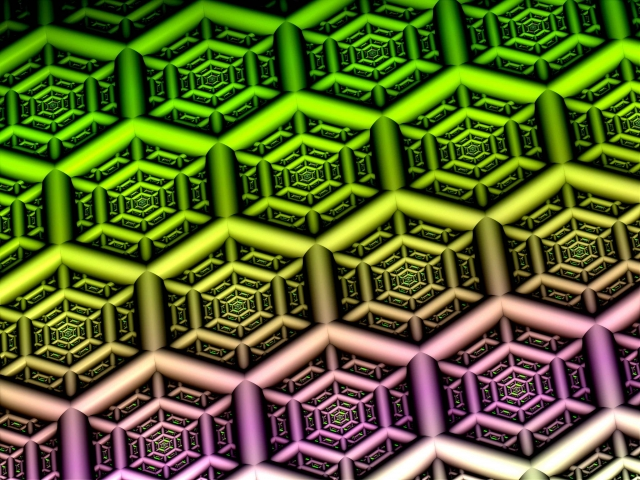Hexagons are essentially 6 equilateral triangles put together at their apexes.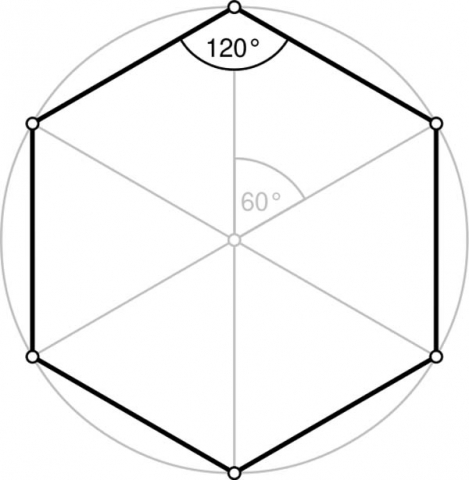The triangle is also the preeminent symbol of divinity. In prayer our arms spontaneously form a three-sided 120 degree corner.The proportions of the sitting Buddha fall within the equilateral triangle.Reference Construction Lesson #22: Triangles – Part 1.

These include:

1. Constructing the equilateral triangle from one circle.
######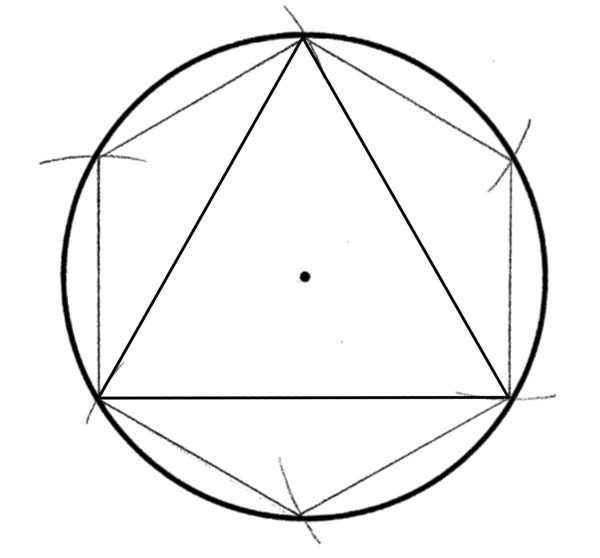1. Constructing the Equilateral triangle from the Vesica Piscis.

As we have learned, there is profound harmony to the Vesica’s space.

The triangle is the initial shape to emerge through it.

The triangle automatically forms from the Vesica by connecting the higher intersection point to the two centers.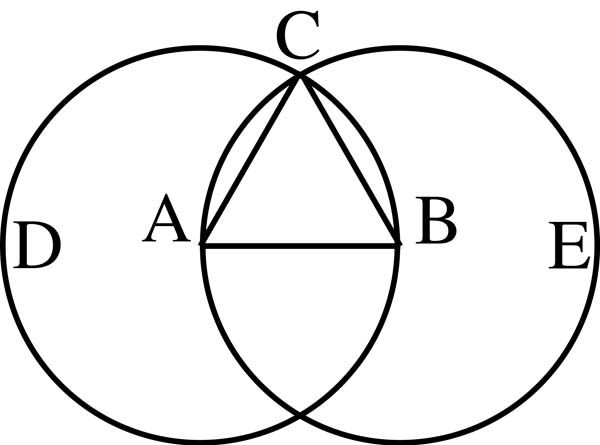Two triangles can be defined. This is the familiar representation of two tetrahedra back-to-back.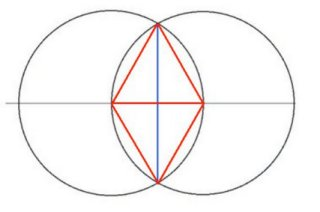One key to sacred geometry is realizing how these perfect shapes form due to the center of one circle being attracted to the center of another circle.

In other words, the infinite source in one attracts to the infinite source in another, yearning to be reunited through the action of gravity/love (the movement of the line).

As you draw sacred geometric patterns, you connect centers and intersections to create all shapes, and everything naturally falls into place without having to measure.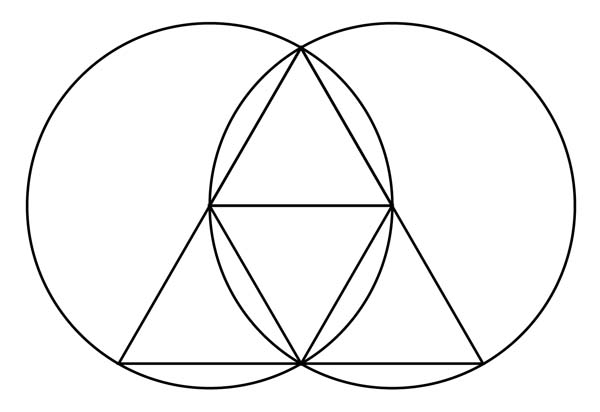“Every triangle we construct is not merely a copy of another person’s triangle but is an approximation of an ideal principle.” 1

1. Three Circles – Triangles

Circles effortlessly produce perfect triangles.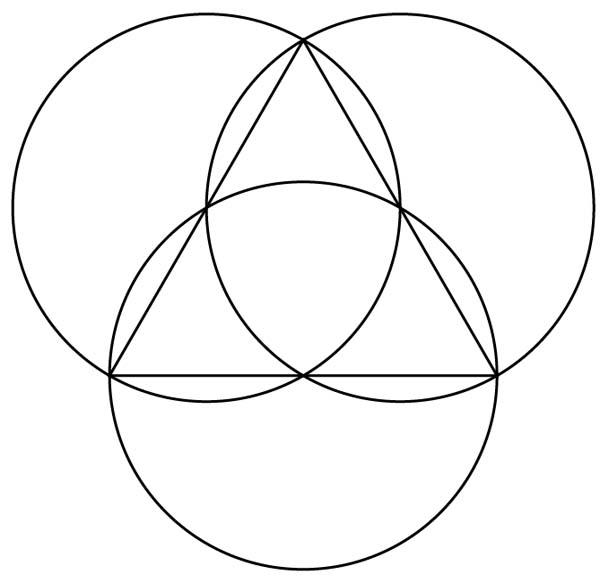1. Three Circles – A Hexagon within the Vesica

From the Vesica, adding a third equal circle defines the six points of a perfect hexagon.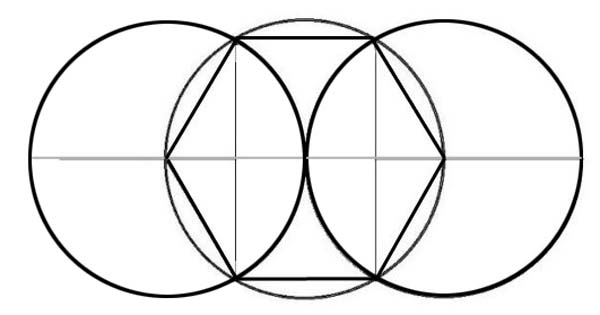Each hexagon contains six equilateral triangles pointing inwards.1. Constructing an equilateral triangle from a Line Segment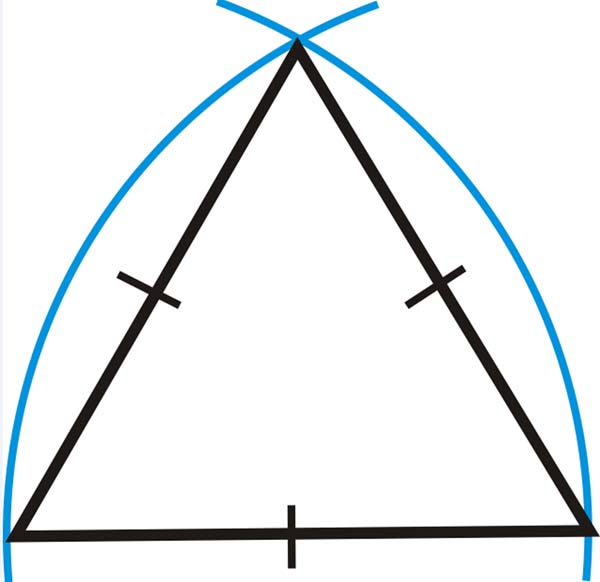1. Ad Triangulum

Ad Triangulum means “of the triangle”.

Ad Triangulum along with Ad Quadratum were the two great systems of Masonic Geometry in use throughout the Middle Ages and Renaissance.

Ad Triangulum is based on the proportions of the equilateral triangle.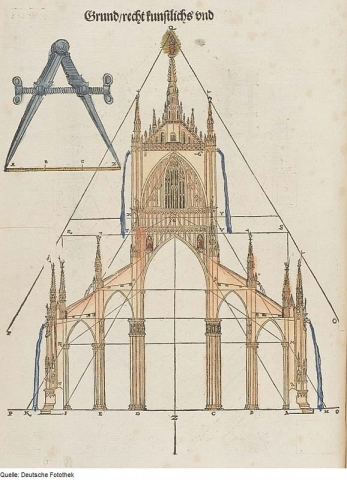An equilateral triangle is cut by its altitude creating two equal right-angled triangles.

Each have side lengths of 1, 2 and √3.

The proportion of 1 : 2 : √3 is the essence of Ad Triangulum.

1 is the base of the equilateral triangle cut in half.

2 is the hypotenuse.

√3 is side that was the altitude of the equilateral triangle.

Randall Carlson comments, “This right triangle, with a hypotenuse double the length of the short side, or leg, is the basic proportioning triangle in the Ad Triangulum system of Masonic geometry. We know that all interior angles of an equilateral triangle equal 60.”

Therefore, we know the angle that was not cut in half is 60º.

The angle opposite the hypotenuse would be 90º.

The third angle will be 30º. (60+90+30 = 180)

This half equilateral triangle – the 30-60-90 triangle – was claimed by Timaeus (Plato) to be the most beautiful in the universe.

Plato allocates this triangle to the construction of the molecules ‘fire’, ‘water’ and ‘air’ corresponding to the tetrahedron, icosahedron, and octahedron.1. Schneider, Michael, A Beginner’s Guide to Constructing the Universe, Harper Perennial, 1994

Return to Free Library

Return to Number Menu